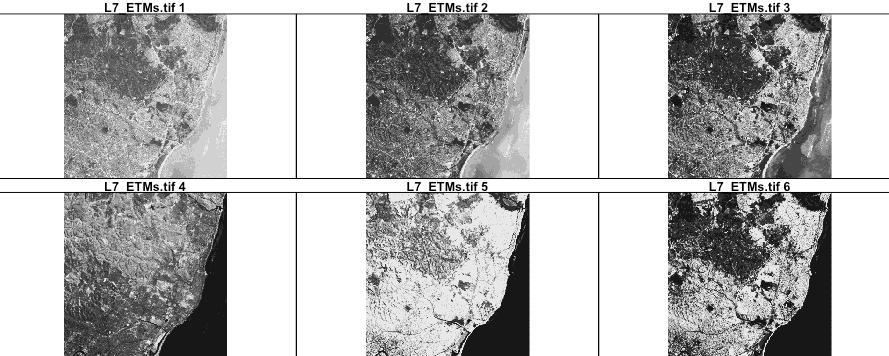/ plotly.R Public

# Special bindings for stars data structures#1200

Open
opened this issue Feb 20, 2018 · 0 comments
Open

# Special bindings for stars data structures #1200

opened this issue Feb 20, 2018 · 0 comments
Labels

 A good start would be to replicate the `plot.stars()` method (from https://github.com/r-spatial/stars) ```library(stars) etm <- st_stars(system.file("tif/L7_ETMs.tif", package = "stars")) plot(etm)```So `plot_ly(etm)` would do something similar to this (automatically) ```library(sf) etm <- st_transform(etm, 3857) band <- st_dimensions(etm)[["band"]] n <- band\$to - band\$from + 1 ann <- list(x = 0.5, y = 1, yanchor = "bottom", xanchor = "middle", xref = "paper", yref = "paper", showarrow = FALSE) plots <- lapply(seq_len(n), function(i) { z <- t(etm[][,,i]) ann\$text <- paste(names(etm), i) plot_ly(z = z[seq.int(nrow(z), 1), ]) %>% layout(annotations = ann) %>% colorbar(title = i) }) subplot(plots, nrows = 2, titleX = TRUE, shareX = TRUE, shareY = TRUE)```The text was updated successfully, but these errors were encountered: